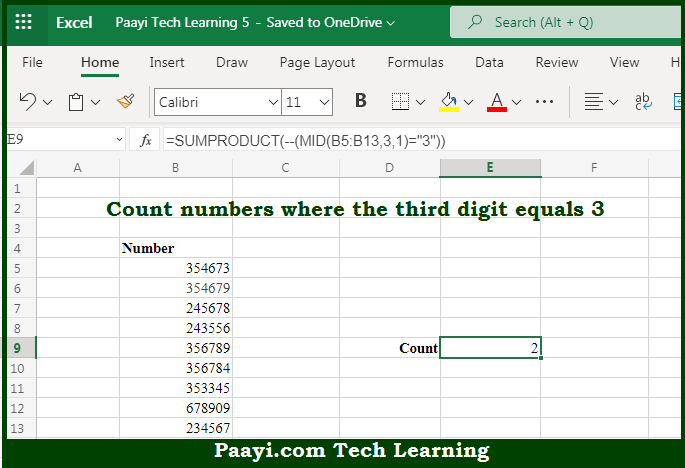# Learn How to Count Numbers Third Digit Equals To 3 in Microsoft Excel

Written by | 0 Comments | 568 Views

In this article, you will learn how to COUNT various things in Microsoft Excel using a single or combination of functions and its purpose in Microsoft Excel. You will also get to know how to Count Numbers Third Digit Equals To 3 & see the generic formula

Count Numbers Third Digit Equals To 3 in Microsoft Excel

The main purpose of this formula is to count numbers where the third digit equals 3. Here we will learn how to count numbers where the third digit is equivalent to numeric value 3 in Microsoft Excel. That implies, with the help of a formula based on the SUMPRODUCT and MID functions you can able to count numbers where the third digit equals 3. So, with the help of this formula, you can able to count numbers where the third digit is equivalent to numeric value 3.

General Formula to Count Numbers Third Digit Equals To 3

=SUMPRODUCT(--(MID(range,3,1)="3"))

The Explanation for the Count Numbers Third Digit Equals To 3So we know that with the help of the given formula above you can able to count numbers where the third digit equals 3. Here we will learn how to count numbers where the third digit is equivalent to numeric value 3 in Microsoft Excel. As we know that, the first argument given here is a cell reference, the second argument specifies the start number, and the third argument indicates the number of characters. In case you use the MID function the range of cells for the first argument, you'll get back an array of results. So, with the help of this formula, you can able to count numbers where the third digit equals 3.